# Formula for the cells on the same address, but on the different sheets

Let us have several identical tables that are on different Excel worksheets, but always on the same address. How to make SUM or AVERAGE of these tables?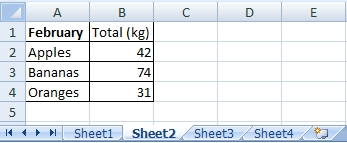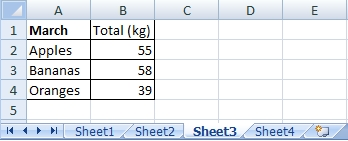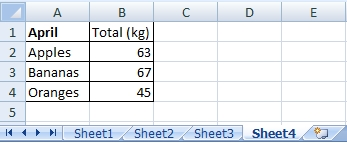I will create SUM and AVERAGE of these tables to the new Sheet5. The formulas for the first item (Apples) are

=SUM(Sheet1:Sheet4!B2)

=AVERAGE(Sheet1:Sheet4!B2)

This formulas include all of the cells B2, which are on the Sheet1, Sheet2, Sheet3 and Sheet4.

If the sheets were named according to months, the formula would look like this:

=SUM(January:April!B2)

## Solution of the SUM function: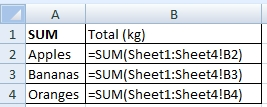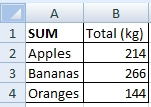## Solution of the AVERAGE function: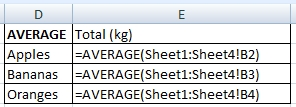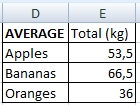Important: It depends on the sheets order in the workbook. If it is like on the following picture, the formula will include only Sheet1, Sheet2 and Sheet4. Excel takes into account the order of the sheets and not their names.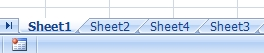1.